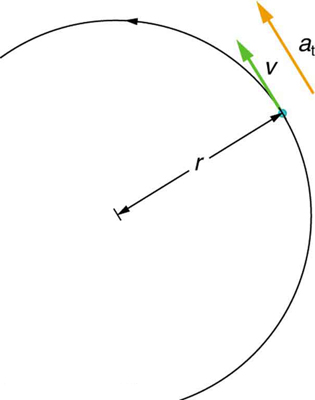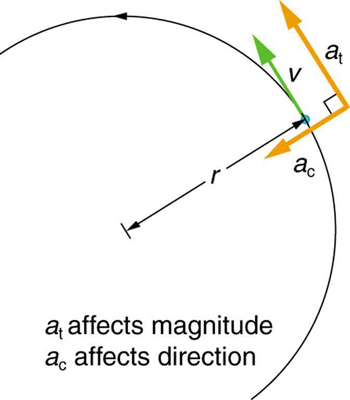# 33 5.3 Angular Acceleration

Angular acceleration is denoted by the Greek letter alpha (α). Angular acceleration represents the time rate of change in angular velocity. Another way to think about this is how quickly something is speeding up or slowing down.

acceleration (α) = Δω/Δt

The units are rads/s2 or degrees/s2. When velocity is increasing, the acceleration is in the same direction of rotation to increase the velocity. When the velocity is decreasing, there has to be acceleration in the opposite direction of travel acting as a break to decrease the velocity.

Acceleration has a direction. If the object is moving in the counterclockwise direction (+) and gaining velocity, acceleration is positive. If velocity is decreased, acceleration is negative. If the object is moving in the clockwise direction (-) and gaining velocity, acceleration is negative. If velocity is decreased, acceleration is positive.

### Example 1: Calculating the Angular Acceleration and Deceleration of a Bike Wheel

Suppose a teenager puts her bicycle on its back and starts the rear wheel spinning from rest to a final angular velocity of 250 rpm in 5.00 s. (a) Calculate the angular acceleration in rad/s2. (b) If she now slams on the brakes, causing an angular acceleration of -87.3 rad/s2, how long does it take the wheel to stop?

Strategy for (a)

The angular acceleration can be found directly from its definition in $\boldsymbol{\alpha=\frac{\Delta\omega}{\Delta{t}}}$ because the final angular velocity and time are given. We see that Δω is 250 rpm and Δt is 5.00 s.

Solution for (a)

Entering known information into the definition of angular acceleration, we get

$\begin{array}{lcl} \boldsymbol{\alpha} & \boldsymbol{=} & \boldsymbol{\frac{\Delta\omega}{\Delta{t}}} \\ {} & \boldsymbol{=} & \boldsymbol{\frac{250\textbf{ rpm}}{5.00\textbf{ s.}}} \end{array}$

Because Δω is in revolutions per minute (rpm) and we want the standard units of rad/s2 for angular acceleration, we need to convert Δω from rpm to rad/s:

$\begin{array}{lcl} \boldsymbol{\Delta\omega} & \boldsymbol{=} & \boldsymbol{250\frac{\textbf{rev}}{\textbf{min}}\cdotp\frac{2\pi\textbf{ rad}}{\textbf{rev}}\cdotp\frac{1\textbf{ min}}{60\textbf{ sec}}} \\ {} & \boldsymbol{=} & \boldsymbol{26.2\textbf{ rads.}} \end{array}$

Entering this quantity into the expression for $\boldsymbol{\alpha}$, we get

$\begin{array}{lcl} \boldsymbol{\alpha} & \boldsymbol{=} & \boldsymbol{\frac{\Delta\omega}{\Delta{t}}} \\ {} & \boldsymbol{=} & \boldsymbol{\frac{26.2\textbf{ rad/s}}{5.00\textbf{ s}}} \\ {} & \boldsymbol{=} & \boldsymbol{5.24\textbf{ rad/s.}^2} \end{array}$

Strategy for (b)

In this part, we know the angular acceleration and the initial angular velocity. We can find the stoppage time by using the definition of angular acceleration and solving for Δt, yielding

$\boldsymbol{\Delta{t}\:=}\boldsymbol{\frac{\Delta\omega}{\alpha}}.$

Solution for (b)

Here the angular velocity decreases from 26.2 rad/s (250 rpm) to zero, so that Δω is -26.2 rad/s, and $\boldsymbol{\alpha}$ is given to be -87.3 rad/s2. Thus,

$\begin{array}{lcl} \boldsymbol{\Delta{t}} & \boldsymbol{=} & \boldsymbol{\frac{-26.2\textbf{ rad/s}}{-87.3\textbf{ rad/s}^2}} \\ {} & \boldsymbol{=} & \boldsymbol{0.300\textbf{ s.}} \end{array}$

Discussion

Note that the angular acceleration as the girl spins the wheel is small and positive; it takes 5 s to produce an appreciable angular velocity. When she hits the brake, the angular acceleration is large and negative. The angular velocity quickly goes to zero. In both cases, the relationships are analogous to what happens with linear motion. For example, there is a large deceleration when you crash into a brick wall—the velocity change is large in a short time interval.

If the bicycle in the preceding example had been on its wheels instead of upside-down, it would first have accelerated along the ground and then come to a stop. This connection between circular motion and linear motion needs to be explored. For example, it would be useful to know how linear and angular acceleration are related. In circular motion, linear acceleration is tangent to the circle at the point of interest, as seen in Figure 2. Thus, linear acceleration is called tangential acceleration at.Figure 2. In circular motion, linear acceleration a, occurs as the magnitude of the velocity changes: a is tangent to the motion. In the context of circular motion, linear acceleration is also called tangential acceleration at.

Linear or tangential acceleration refers to changes in the magnitude of velocity but not its direction. We know that in circular motion centripetal acceleration, ac, refers to changes in the direction of the velocity but not its magnitude. An object undergoing circular motion experiences centripetal acceleration, as seen in Figure 3. Thus, at and ac are perpendicular and independent of one another. Tangential acceleration at is directly related to the angular acceleration $\boldsymbol{\alpha}$ and is linked to an increase or decrease in the velocity, but not its direction.Figure 3. Centripetal acceleration ac occurs as the direction of velocity changes; it is perpendicular to the circular motion. Centripetal and tangential acceleration are thus perpendicular to each other.

Now we can find the exact relationship between linear acceleration at and angular acceleration $\boldsymbol{\alpha}$. Because linear acceleration is proportional to a change in the magnitude of the velocity, it is defined to be

$\boldsymbol{a_{\textbf{t}}\:=}\boldsymbol{\frac{\Delta{v}}{\Delta{t}}.}$

For circular motion, note that v=rω, so that

$\boldsymbol{a_{\textbf{t}}\:=}\boldsymbol{\frac{\Delta(r\omega)}{\Delta{t}}.}$

The radius r is constant for circular motion, and so Δ(rω)=r(Δω). Thus,

$\boldsymbol{a_{\textbf{t}}=r}\boldsymbol{\frac{\Delta\omega}{\Delta{t}}.}$

By definition, $\boldsymbol{\alpha=\frac{\Delta\omega}{\Delta{t}}}.$ Thus,

$\boldsymbol{a_{\textbf{t}}=r\alpha},$

or

$\boldsymbol{\alpha\:=}\boldsymbol{\frac{a_{\textbf{t}}}{r}.}$

These equations mean that linear acceleration and angular acceleration are directly proportional. The greater the angular acceleration is, the larger the linear (tangential) acceleration is, and vice versa. For example, the greater the angular acceleration of a bike’s drive wheels, the greater the acceleration of the bike. The radius also matters. For example, the smaller a wheel, the smaller its linear acceleration for a given angular acceleration $\boldsymbol{\alpha}$.

So far, we have defined three rotational quantities— θ, ω, and $\boldsymbol{\alpha}$. These quantities are analogous to the translational quantities x, v, and a. Table 1 displays rotational quantities, the analogous translational quantities, and the relationships between them.

Rotational Translational Relationship
$\boldsymbol{\theta}$ $\boldsymbol{x}$ $\boldsymbol{\theta=\frac{x}{r}}$
$\boldsymbol{\omega}$ $\boldsymbol{v}$ $\boldsymbol{\omega=\frac{v}{r}}$
$\boldsymbol{\alpha}$ $\boldsymbol{a}$ $\boldsymbol{\alpha=\frac{a_{\textbf{t}}}{r}}$
Table 1. Rotational and Translational Quantities.

# Section Summary

• Uniform circular motion is the motion with a constant angular velocity $\boldsymbol{\omega=\frac{\Delta\theta}{\Delta{t}}}.$
• In non-uniform circular motion, the velocity changes with time and the rate of change of angular velocity (i.e. angular acceleration) is $\boldsymbol{\alpha=\frac{\Delta\omega}{\Delta{t}}}.$
• Linear or tangential acceleration refers to changes in the magnitude of velocity but not its direction, given as $\boldsymbol{a_{\textbf{t}}=\frac{\Delta{v}}{\Delta{t}}}.$
• For circular motion, note that v=rω, so that
$\boldsymbol{a_{\textbf{t}}\:=}\boldsymbol{\frac{\Delta(r\omega)}{\Delta{t}}}.$
• The radius r is constant for circular motion, and so Δ(rω)=rΔω. Thus,
$\boldsymbol{a_{\textbf{t}}=r}\boldsymbol{\frac{\Delta\omega}{\Delta{t}}}.$
• By definition, Δω/Δt=$\boldsymbol{\alpha}$. Thus,
$\boldsymbol{a_{\textbf{t}}=r\alpha}$

or

$\boldsymbol{\alpha=}\boldsymbol{\frac{a_{\textbf{t}}}{r}}.$

### Problems & Exercises

4: Unreasonable Results

You are told that a basketball player spins the ball with an angular acceleration of 100 rad/s2. (a) What is the ball’s final angular velocity if the ball starts from rest and the acceleration lasts 2.00 s? (b) What is unreasonable about the result? (c) Which premises are unreasonable or inconsistent?

## Glossary

angular acceleration
the rate of change of angular velocity with time
change in angular velocity
the difference between final and initial values of angular velocity
tangential acceleration
the acceleration in a direction tangent to the circle at the point of interest in circular motion

### Solutions

Problems & Exercises

4: